## Monte-Carlo Simulation with Risk Kit

### Contents

1.  The need for rapid-prototyping of Monte-Carlo simulations

2.  Presentation of Risk Kit

3.  A profit and loss simulation

3.1.  The deterministic starting point

3.2.  The sources of randomness

3.3.  Defining the model outputs

3.4.  Configure the simulation

3.5.  Run the simulation

4.  Plots

4.1.  Statistics functions

5.  Sensitivity analysis

5.1.  Sensitivity analysis via Risk Kit cell-function

6.  Conclusion

7.  Our Products

## 1.The need for rapid-prototyping of Monte-Carlo simulations

To top

Monte-Carlo simulations play an increasing role in finance, social sciences and risk management. Monte-Carlo simulations are a generic technique to estimate the distributions of arbitrary target quantities in complex environments. Among those are profit distributions, shareholder value distributions, portfolio loss distributions and generally the distribution of any target quantity that is subject to hazard.

However, to use Monte-Carlo methods in practice is often subject to severe difficulties such as the need to draw random variates, to program a model and to evaluate the simulation results. This requires the handling of advanced mathematical methods as well as of modern computer technology.

Risk Kit is a tool for the rapid-prototyping of risk analyses and Monte-Carlo simulations. It provides the components of quantitative risk management and allows linking them in a simple and intuitive way to complete models and risk analyses. Risk Kit allows users to efficiently access quantitative risk analyses and Monte-Carlo simulations. It accelerates the development time many times over as compared to conventional approaches.

All Risk Kit functions can be integrated in spreadsheet analyses as Microsoft Excel cell-functions. Moreover, Risk Kit functions can be called from VBA or the .NET programming language. This way Risk Kit can be integrated in a production environment.

This document describes how to perform Monte-Carlo simulations and risk analyses with Risk Kit1.

## 2.Presentation of Risk Kit

To top

Risk Kit is an add-in for Microsoft Excel. After installation it is available in the ‘Risk Kit’ toolbar in Excel (see Figure 1).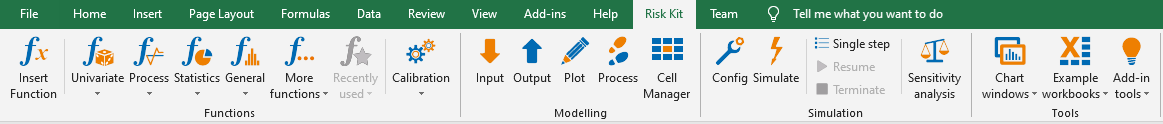Figure 1 - The Risk Kit toolbar in Microsoft Excel

Moreover, the cell context menu now includes the ‘Risk Kit’ sub-menu that duplicates the Risk Kit toolbar (see Figure 2).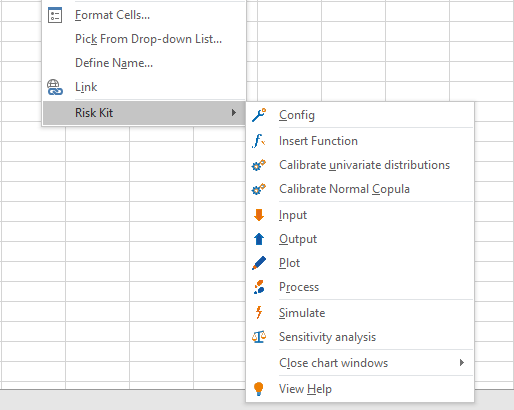Figure 2 - Cell context menu

Table 1 describes all buttons from the Risk Kit toolbar.

Table 1 - Description of the Risk Kit toolbar

 Button Description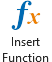Opens the Function dialog that allows to choose Risk Kit cell-functions.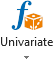Opens the gallery of univariate distributions that allows to select a distribution to draw random variates or calculate the density, mass function, distribution function or inverse cumulative distribution function.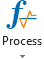Opens the list of functions for stochastic processes.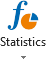Opens the list of statistic functions.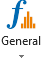Opens the list of general functions, for example to create plots.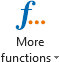Opens additional functions grouped by multivariate distributions, rating methods and fixed income.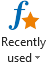Opens recently used functions.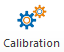Opens the Calibration dialog that allows to calibrate univariate and multivariate distributions to empirical data.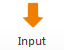Marks a cell as Input-cell.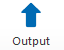Marks a cell as Output-cell.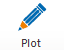Marks a cell as Plot-cell.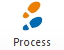Marks a cell as Process-cell.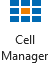Opens the cell manager to show Risk Kit-function cells in open workbooks.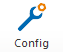Opens the configuration dialog that allows the setting of simulation parameters, changing of the user interface language and others.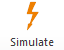Starts the simulation.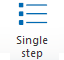Makes a single step of the simulation.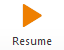Resumes the simulation. The button is available if the simulation is paused.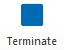Aborts the simulation. The button is available if the simulation is paused.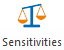Opens the Sensitivity Analysis Form that allows making the sensitivity analysis.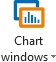The button represents a drop-down list that consists of six items:‘Arrange chart windows’ – Arranges the windows so that all charts are visible at once;‘Cascade chart windows’ – Cascades chart windows one behind the other;‘Close chart windows’ – Closes all chart windows;‘Delete charts from active worksheet’ – Deletes embedded charts from the active worksheet;‘Delete charts from active workbook’ – Deletes embedded charts from the active workbook;‘Delete charts from all workbooks’ – Deletes embedded charts from all open workbooks.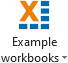Contains a variety of example workbooks to quickly get started with Risk Kit.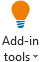Opens the Risk Kit add-in tools to open the online help, change the system language between English, French and German, create key-board shortcuts, send feedback to the Risk Kit developers, recommend Risk Kit to new users, solve problems and get information on the Risk Kit version used.

To do a Monte-Carlo simulation with Risk Kit of a hitherto deterministic model, one can proceed in four steps. Firstly, one or more inputs of the model have to be identified that become random variables in the simulation. Their previously deterministic value will be replaced by random variates that change their value in each simulation run. Through these variables randomness enters the model.

Secondly, the model outputs or target quantities need to be defined. They are calculated from the random inputs and maybe other deterministic inputs in the context of the model.

Thirdly, a number of simulation runs needs to be chosen so that, fourthly, the simulation can be started.

## 3.A profit and loss simulation

To top

### 3.1.The deterministic starting point

To give an example of how to turn a standard deterministic model into a Monte-Carlo simulation, we consider a basic profit and loss model.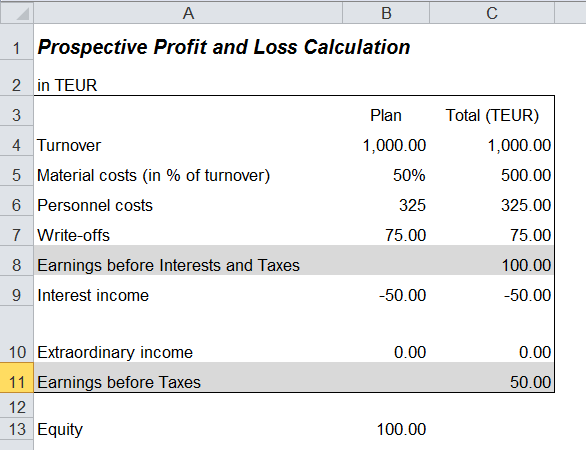Figure 3 – Deterministic profit and loss calculation

To calculate the profit and loss, we simply start with the company’s turnover (C4) and deduct all costs. In a first step, these are material (C5) and personnel costs (C6) and write-offs (C7) that lead to the intermediate result ‘Earnings before interests and taxes’ (EBIT) (C8). Further on the interest income (C9) and the extraordinary income (C10) are deducted from this figure to give the ‘Earnings before taxes’ (EBT) (C11) or the gross profit and loss. All formulas are simple sums and differences.

Since the actual material costs are largely variable and depend on the level of turnover, we model them as a relative quantity ‘in % of turnover’. Therefore, the total material costs in C5 = B5 * C4.

### 3.2.The sources of randomness

To top

After having defined the deterministic model, the first step to turn it into a Monte-Carlo simulation is to identify the sources of randomness in the model.

We suppose that the turnover, the material costs, the personnel costs and the extraordinary income are subject to risks (column B). The aim is to analyze their impact on total EBIT and EBT (column C). Note that risks can also occur as opportunities (e.g. random cost reductions) leading to an increase in income.

While a turnover of 1,000 TEUR was supposed in the deterministic model, we know by experience that this quantity is not stable, but that it fluctuates in practice between 900 and 1050 TEUR with 1000 TEUR being the likeliest amount. Thus, we choose the triangular distribution as an intuitive model for the fluctuation of the turnover.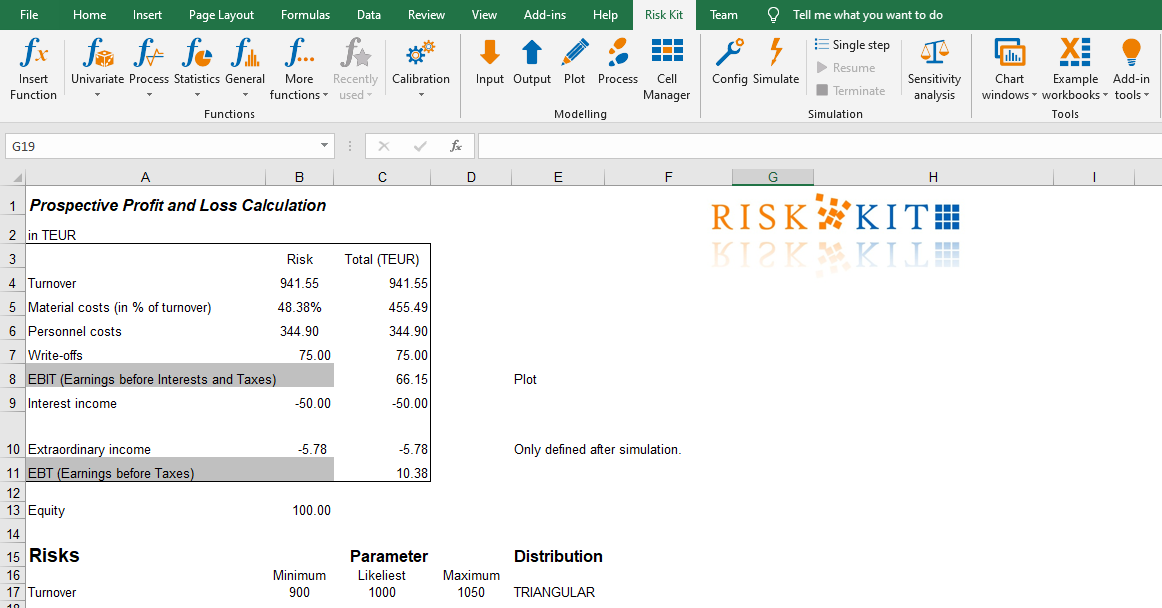Figure 4 - Modelling fluctuations of the turnover

To enter a triangular random variate into the model, we mark cell B4 and click the ‘Insert Function’-button on the Risk Kit toolbar.

Then this dialog appears: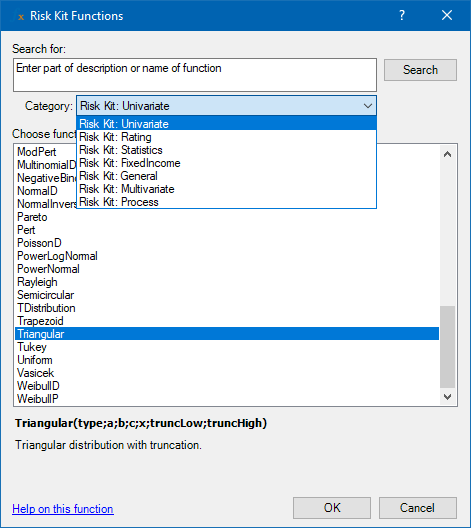Figure 5 - Functions dialog

Risk Kit provides more than 430 cell-functions in 7 categories:

1. Univariate - Univariate probability distributions;

2. Multivariate - Multivariate probability distributions. This list contains functions that supply random variates from standard multivariate distributions as well as from copula functions;

3. Process - Trends and random paths and in some cases also point wise percentiles of stochastic processes;

4. Statistics - Functions for calculating statistics of simulated distributions such as mean, variance, percentiles etc.;

5. General - Functions to plot arbitrary probability distributions and stochastic processes, a function for dynamic sorting, functions for portfolio optimisation and calculation of the efficient frontier, and functions implementing delta-gamma engines;

6. FixedIncome - Functionalities related to interest rate products including the handling of interest rate and spread curves, the calculation of forward rates, the calculation of cash flows for interest bearing products such as loans and bonds, the valuation of fixed and floating rate bonds and the calculation of default probabilities implied in bond spreads;

7. Rating - Rating functions for small and medium sized companies in Germany, Austriaand the US.

Since we want to enter a triangular random variate, we choose the category ‘Univariate’ and select the item Triangular from the list.

Having confirmed with ‘OK’, six functions are displayed: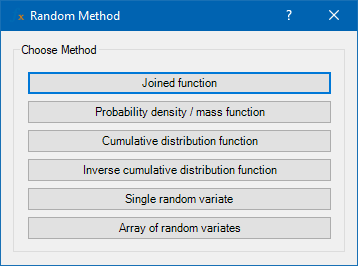Figure 6 - Univariate distribution functions

1. A joined function where the function type is chosen through a parameter.

2. The probability density function (PDF) in case of continuous distributions or the probability mass function (PMF) in case of discrete distributions.

3. The cumulative distribution function (CDF) and

4. The inverse cumulative distribution function (ICDF) and

5. Single random variate from the respective distribution and

6. Array of random variates from the respective distribution. These functions are used in the ‘High speed’ simulation mode.

These functions are available for each univariate distribution.

Click ‘Single random variate’ to get a random number of the triangular distribution.

An alternative and often more intuitive way to select a distribution is to click the button ‘Univariate’ on the toolbar and open the distribution gallery.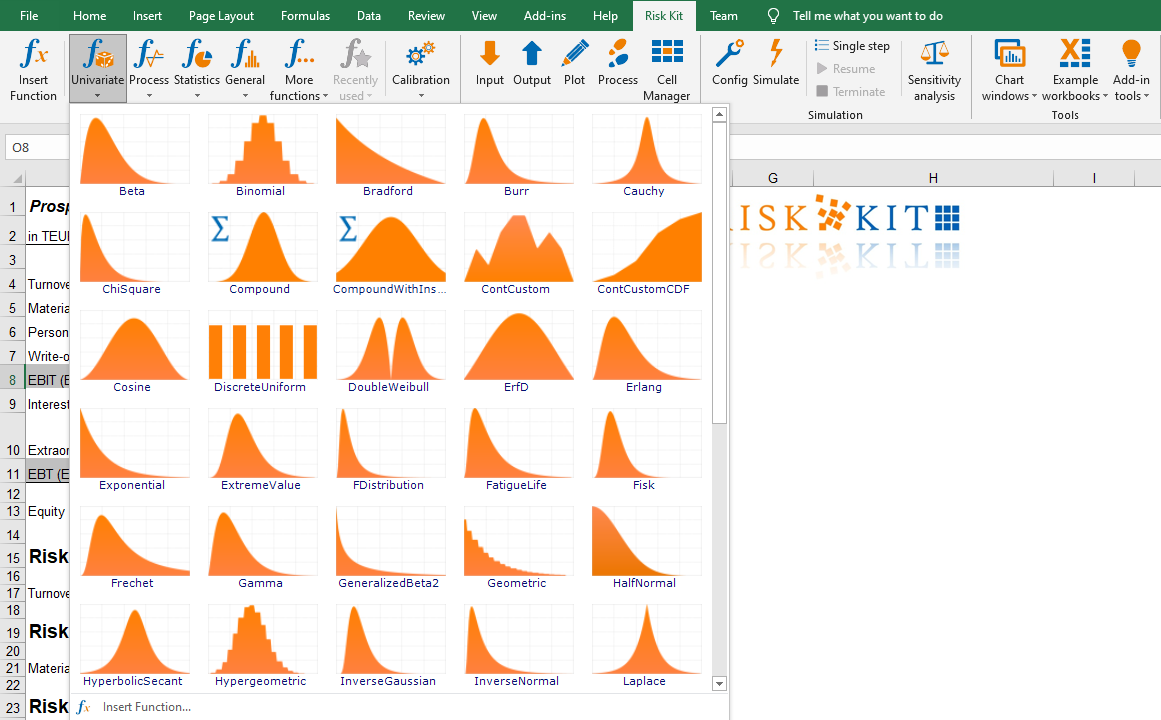Figure 7 - Distribution gallery

The gallery shows a preview icon for each distribution that illustrates a typical shape of the distribution’s density or mass function. The distributions are also ordered by name.

Hover the mouse cursor over a preview icon to get more information on the distribution. Also, a keyboard-shortcut to the distribution is displayed if one has been defined2.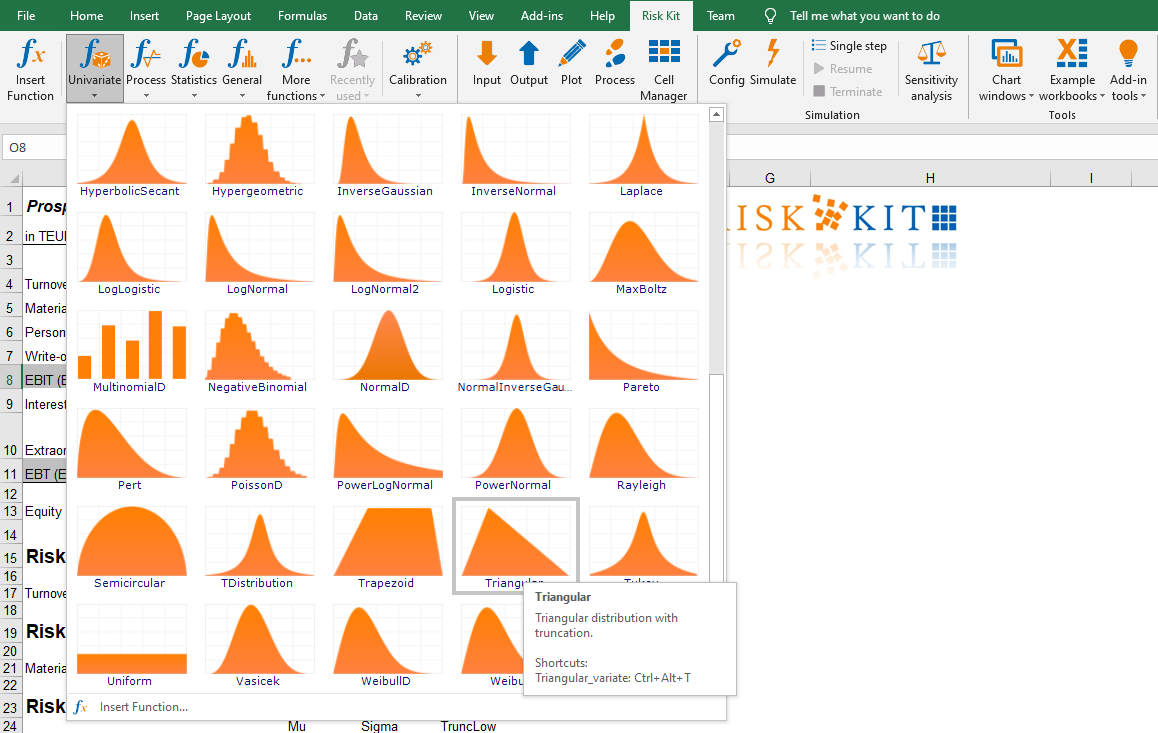Figure 8 - Distribution and shortcut information in the gallery

Click on a distribution to select it from the gallery and choose ‘Single random variate’ as described above.

You can now enter the distribution parameters.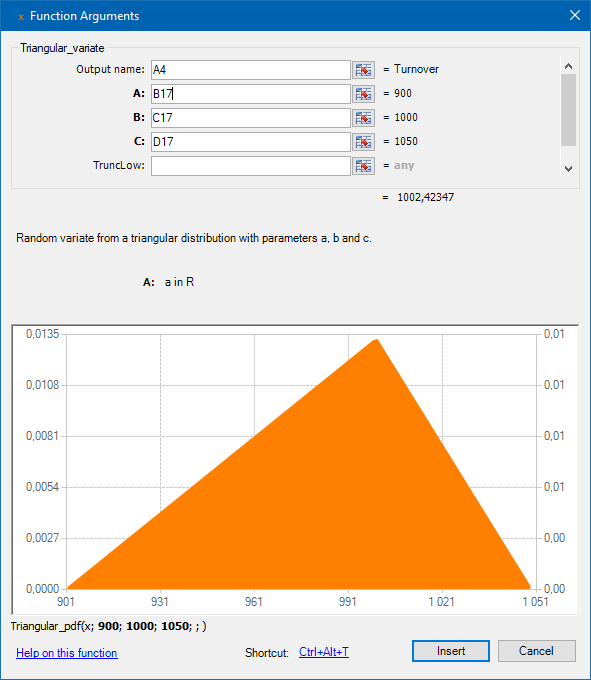Figure 9 – Function arguments of the triangular distribution

There are two ways to do so. You can either store the parameters in the Excel sheet and reference the respective cells. This is displayed in figure 9 where the parameter ‘a’ contains a reference to cell B17 with the most pessimistic turnover supposed, ‘b’ contains a reference to C17 with the likeliest and ‘c’ to D17, the most optimistic turnover supposed.

Alternatively, you could enter the parameters directly.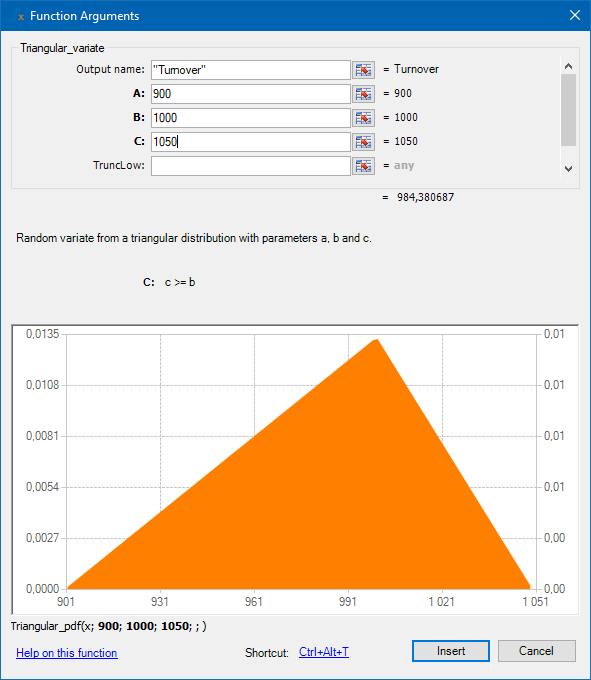Figure 10 – Function arguments

The dialog allows you to specify an output name (for univariate distributions only). The output name will appear as legend for this distribution in charts and statistics outputs.

The dialog also offers you further information on the chosen function and its parameters. Firstly, you get a brief summary of what the function does. In this case it produces ‘random variates from a triangular distribution with parameters a, b and c’.

When you enter a particular parameter, information on its range of definition is displayed. In our case the parameter c is required to be greater or equal to b.

Below the chart you see the definition of the function that is actually plotted.

Finally, note the link to the online help in the lower left corner of the dialog and the function shortcut at the bottom.

By clicking on ‘Insert’, the function definition is entered into cell B4 and appears in Excel’s formula bar. Note that the total turnover in C4 now reflects the risk. Also, the material costs are already affected albeit their percentage is still constant at 50% since they also depend on the turnover.

You can manually redraw random variates by pressing <F9>.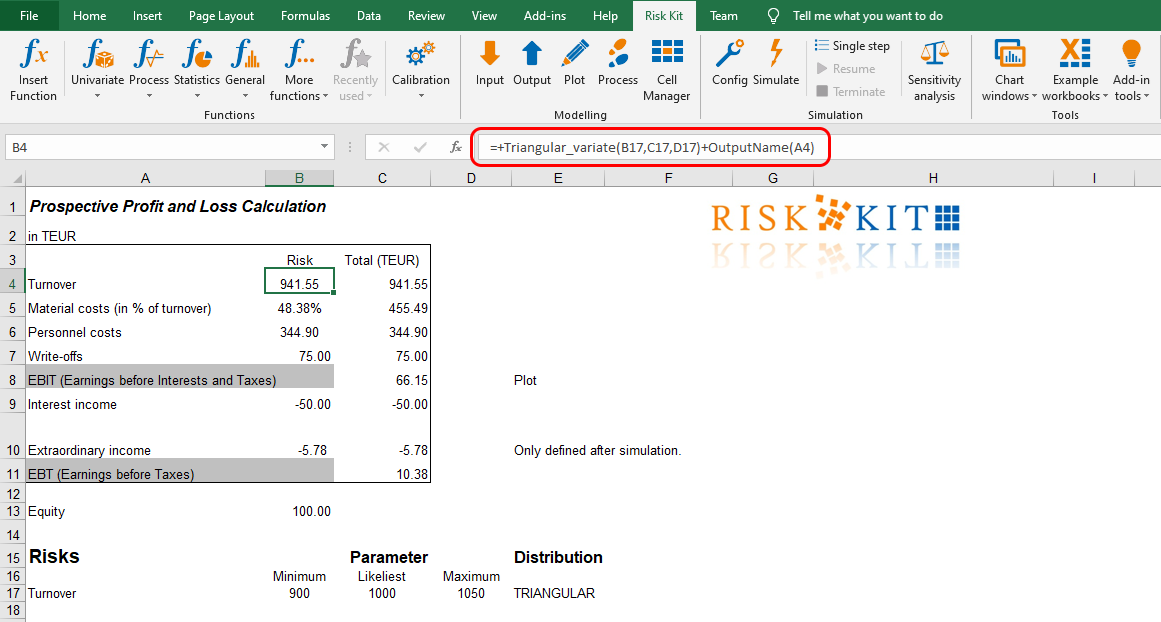Figure 11 – Cell containing a Risk Kit function

We proceed similarly for the other risks. For the relative deviation from the planned material costs, we assume a normal distribution with expectation mu = 50% and standard deviation sigma = 3%.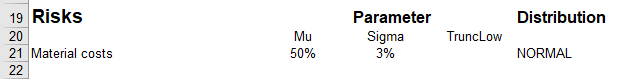Figure 12 - Model assumption for the material costs

To enter the formula into cell B5, we place the cursor on this cell and open the Risk Kit function dialog. In the field ‘Search for:’ we enter ‘normal’ and confirm with ‘Search’ or press Enter. The result delivers all Risk Kit functions related to anything ‘normal’. If we mark a function, we get a brief explanation of its meaning. We, thus, find that the function ‘NormalD’ is what we are looking for, the normal distribution.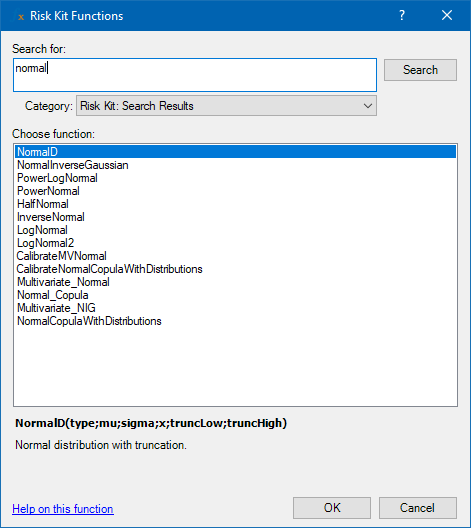Figure 13 - The function search dialog

Confirming with ‘OK’ and choosing ‘Single random variate’ leads us to the form where we can enter the references to B21 for mu and to C21 for sigma. ‘Insert’ enters the function definition into B5. Note that you can abbreviate this process by writing or copying the function definition into the cell right away. The function dialog is just a supporting tool.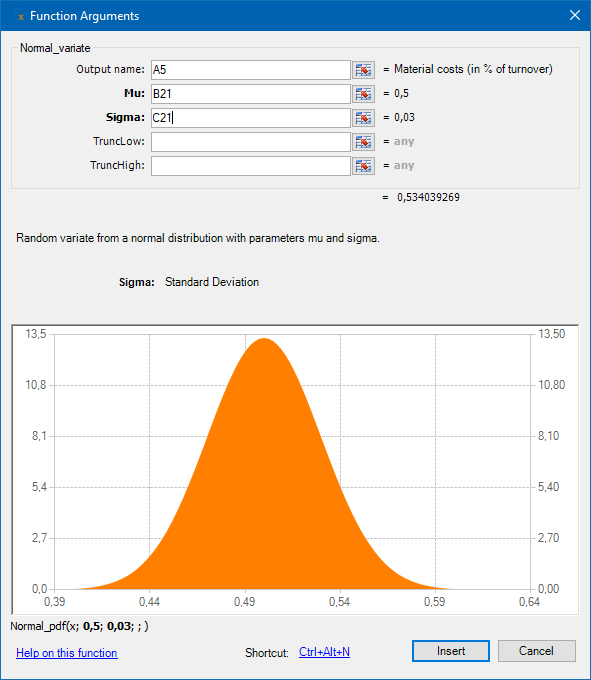Figure 14 - Function arguments for the normal distribution

The material costs in absolute terms are then the simulated costs in % of the turnover times the simulated turnover.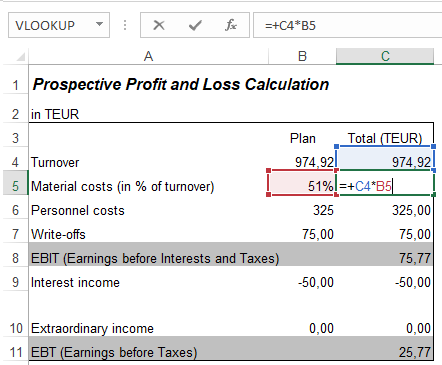Figure 15 - Linking the simulated material costs to the model

The personnel costs we model similar to the material costs. We assume a normal distribution with expectation 325 and standard deviation 20. However, we believe that personnel costs cannot be reduced easily below a certain level. We, thus, assume that they are truncated on the left-hand side at 300.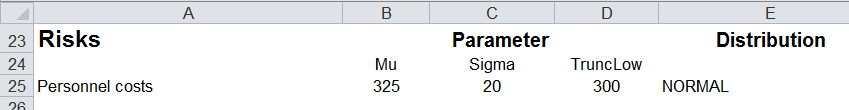Figure 16 - Model assumption for the personnel costs

To turn this assumption into a function call, we open the function dialog and link the parameters mu, sigma and TruncLow to cells B25, C25 and D25, respectively.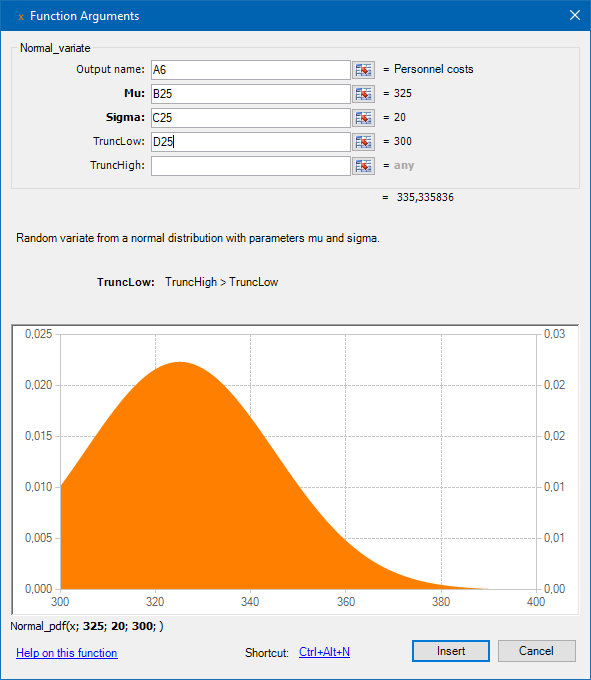Figure 17 - Normal distribution with truncation

The truncation of the distribution at 300 makes sure that no random variates are drawn below this level while on the right-hand side the